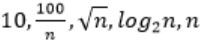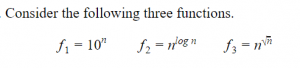## Asymptotic-Complexity

 Question 1

Consider the following functions from positives integers to real numbersThe CORRECT arrangement of the above functions in increasing order of asymptotic complexity is:

 ABCDAlgorithms       Asymptotic-Complexity       GATE 2017 [Set-1]       Video-Explanation
Question 1 Explanation:
In this problem, they are expecting to find us “increasing order of asymptotic complexity”.
Step-1: Take n=2048 or 211 (Always take n is very big number)
Step-2: Divide functions into 2 ways
1. Polynomial functions
2. Exponential functions
Step-3: The above functions are belongs to polynomial. So, simply substitute the value of n,
First compare with constant values.
→ 100 / 2048 = 0.048828125
→ 10 > 100/ 2048
→ log2 2048 =11
→ √n = 45.25483399593904156165403917471
→ n = 2048
So, Option B is correct
 Question 2

Which of the given options provides the increasing order of asymptotic complexity of functions f1, f2, f3 and f4?

`f1(n)=2n     f2(n)=n3/2     f3(n)=n log2 n     f4(n)=nlog2n `
 A f3, f2, f4, f1 B f3, f2, f1, f4 C f2, f3, f1, f4 D f2, f3, f4, f1
Algorithms        Asymptotic-Complexity       GATE 2011
Question 2 Explanation:
If they are expecting to find an asymptotic complexity functions means
→ Divide functions into 2 categories
1. Polynomial functions
2. Exponential functions
Above 4 functions we have only one exponential function is f1 (n) = 2n . So, It’s value is higher than to rest of the functions.
Substitute log on both sides then we get an ascending order is f3, f2, f4.
 Question 3

An array A contains n integers in locations A,A, …………… A[n-1]. It is required to shift the elements of the array cyclically to the left by K places, where 1≤K≤n-1. An incomplete algorithm for doing this in linear time, without using another is given below. Complete the algorithm by filling in the blanks. Assume all variables are suitably declared.

``` min:=n;
i=0;
while _____do
begin
temp:=A[i];
j:=i;
while _____do
begin
A[j]:=_____;
j:=(j+K) mod n;
if j```
 A Theory Explanation.
Algorithms       Asymptotic-Complexity       GATE 1994
 Question 4

Consider the following recursive function:

``` function fib (1:integer);integer;
begin
if (n=0) or (n=1) then fib:=1
else fib:=fib(n-1) + fib(n-2)
end; ```

The above function is run on a computer with a stack of 64 bytes. Assuming that only return address and parameter and passed on the stack, and that an integer value and an address takes 2 bytes each, estimate the maximum value of n for which the stack will not overflow. Give reasons for your answer.

 A Theory Explanation.
Algorithms       Asymptotic-Complexity       GATE 1994
 Question 5Which one of the following options arranges the functions in the increasing order of asymptotic growth rate?
 A f2, f3, f1 B f3, f2, f1 C f2, f1, f3 D f1, f2, f3
Algorithms       Asymptotic-Complexity       GATE 2021 CS-Set-1       Video-Explanation
Question 5 Explanation:

The asymptotic notation order should be

Constant < logarithmic < linear < polynomial < exponential < factorial

F2 and F3 → Polynomial

F1 → Exponential

By the order of asymptotic notations F1 is definitely larger.

Method-1:

Consider n=100

F1 : 100 ^100 ⇒ 1.e+200

F2 : N^log(100) base 2 ⇒  100 ^ log(100) base 2 ⇒ 100^6.6438561897747 = 1,93,96,00,91,15,564.181300016469223466

F3 : N^Sqrt(n) ====> 100^Sqrt(100) ⇒ 100^10 ⇒ 10,00,00,00,00,00,00,00,00,000

Method-2:

We can apply "log" on both sides.

log(F1)=nlog10 (base 2)

log(F2)=(logn)^2 = logn * logn (base 2)

log(F3)=sqrt(n)logn (base 2)

 Question 6
Which one of the following statements is TRUE for all positive functions f (n) ?
 A f ( n ^2 ) ( f ( n ) ^2 ), when f ( n ) is a polynomial B f ( n ^2 ) o ( f ( n ) ^2 ) C f ( n ^2 ) O ( f ( n ) ^2 ), when f ( n ) is an exponential function DAlgorithms       Asymptotic-Complexity       GATE 2022       Video-Explanation
Question 6 Explanation:
constant < logarithmic < linear < polynomial < exponential < factorial
f(n)=n^c where c is a constant
f(n^2) = (n^2)^c = n^2c
(f(n))^2 = (n^c)^2 = n^2c
f(n^2) = (f(n))^2 is TRUE asymptotically.
Option-B: FALSE: The small omega function indicates the tightest upper bound.
f(n)^2 < o(f(n)^2) is FALSE asymptotically.
Option-C: FALSE: Consider f(n)=logn
f(n^2)=logn^2 = 2*logn
(f(n))^2 = (logn)^2 = logn * logn
f(n^2) <=Ω(f(n))^2 is FALSE asymptotically.
Option-D: FALSE:
Exponential values(Eg: 2^n, 3^n,..,k^n where k is a constant ”
f(n)=3^n
f(n^2)=f(3^(n^2)
(f(n))^2 = (3^n)^2 = 3^2n
f(n^2) >= O(f(n))^2 is FALSE asymptotically.
 Question 7
Consider the following recurrence:Then, which of the following statements is/are TRUE?
 A f (2^ n -1) 2^ n -1 B f (2 ^n ) 1 C f (5 . 2 ^n ) 2^ n + 1 1 D f (2^ n + 1) 2 ^n + 1
Algorithms       Asymptotic-Complexity       GATE 2022       Video-Explanation
Question 7 Explanation:
We can solve this problem by eliminating options. Substitute n=1024
Based on the “n” value we can get Option-A, B and C are correct.
 Question 8
Complexity of kruskal's algorithm for finding minimum spanning tree of an undirected graph containing n vertices and m edges if the edges are sorted is:
 A O(mn) B O(m) C O(m+n) D O(n)
Algorithms       Asymptotic-Complexity       Nielit Scientist-B IT 4-12-2016
Question 8 Explanation:
Implementation of Kruskal's algorithm should be implemented in 2 steps:
Step1: Sorting of edges takes O(ELogE) time.
Step2: After sorting, we iterate through all edges and apply find union algorithm.
The find and union operations can take at most O(1) time if you use Disjoint set . So overall complexity is O(ELogE + E) time.
Given the edges are already sorted, so we need to do only second step i.e.,we iterate through all edges and apply find-union algorithm. The find and union operations can take at most O(1) time. So, the total time complexity will be O(E).
 Question 9
You are given the postorder traversal,p, of a binary search tree on the n elements 1,2,..,n. You have to determine the unique binary search tree has P as its postorder traversal. What is the time complexity of the most efficient algorithm for doing this?
 A O(logn) B O(n) C O(nlogn) D none of the above, as the tree cannot be ​ be uniquely determined.
Algorithms       Asymptotic-Complexity       Nielit Scientist-C 2016 march
Question 9 Explanation:
Last element in post order is the root of tree- find this element in inorder-log n time. Now as in quick sort consider this as pivot and split the post order array into 2. All elements smaller than pivot goes to left and all elements larger than pivot goes to right and suppose we have k elements smaller than pivot, these elements will be same in both in-order as well as post order. Now, we already got the root, now left child is the left split and right child is the right split.
T(n) = T(k) + T(n-k-1) + logn
In worst case T(n) = O(nlogn), when k=0
But searching for an element in the in-order traversal of given BST can be done in O(1) because we have n elements from 1...n so there is no need to search an element- if last element in post order is say 5 we take it as root and since 4 elements are split the post order array into two (first 4 elements), (6th element onward) and solve recursively. Time complexity for this would be
T(n) = T(k) + T(n-k-1)+O(1)
Which gives T(n) = O(n)
since we know that all elements must be traversed at least once T(n) = θ(n)
There are 9 questions to complete.

Register Now